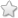# TNSB - 8 - Maths :: Life Mathematics - Term 3

## Course Content / Subtopic list:

1. Introduction To Square Numbers, Summing Up Odd Numbers From One Results In A Square, Summing Up Two Consecutive Natural Numbers Results In A Square,
2. Finding The Square Of A Number Using Diagonal Method, Pythagorean Triplets, Square Root
3. Square Root By Repeated Subtraction Of Successive Odd Numbers, Square Root Through Prime Factorisation, Finding The Square Root Of A Number By Long Division Method
4. Number Of Digits In The Square Root Of A Perfect Square Number, Square Root Of Decimal Numbers, Square Root Of Product And Quotient Of Numbers
5. Approximating Square Roots, Cubes And Cube Numbers, Properties Of Cubes Of Numbers:
6. Cube Root, Cube Root Of A Given Number By Prime Factorisation, Miscellaneous Examples
7. Exponents And Powers- Powers With Positive Exponents, Powers With Zero And Negative Exponents, Expanded Form Of Numbers Using Exponents
8. Laws Of Exponents, Quotient Law, Power Law, Standard Notation And Scientific Notation, Miscellaneous And Practice Problems
0.00(0 votes)